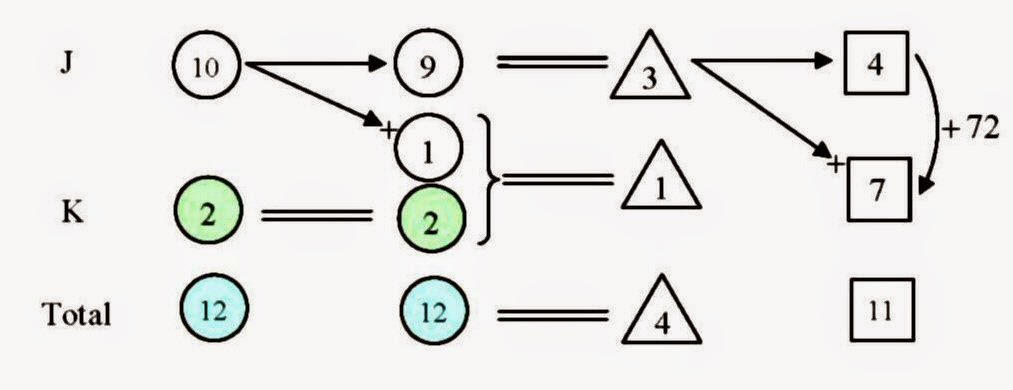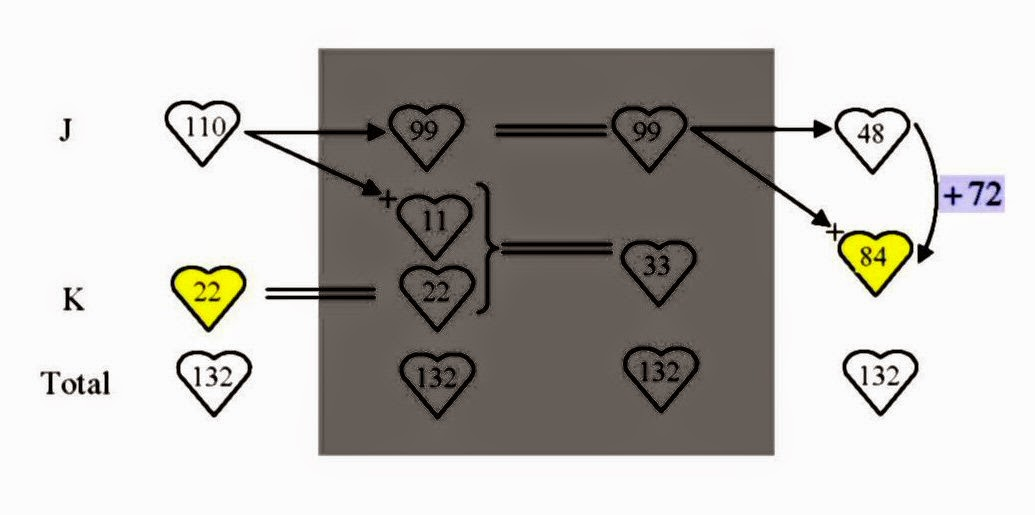## Sunday, March 8, 2015

### [Pri20150306COH] The Cards of Hearts?

Question

Introduction

For this question, I shall illustrate my technique of Distinguished Ratio Units to model the situation.  Read the question carefully and translate the information into a diagram [ using heuristic H02 ] like below:-

I used three different shapes (circle, triangle and square) to envelop the numerical counts of the different kinds ratio units. Although we do not know how many circle units’ worth of cards Kelly had at first, we quickly notice that  9 circle units are equivalent to 3 triangle units, so that one triangle unit is the same as 3 circle units.  So Kelly had 2 circle units’ worth of cards [ heuristic H05 ], as depicted below:-This allows us to answer part (a) of the question already, namely that the required ratio is 10 : 2 i.e. 5 : 1.  With different types of units, it is difficult to compare things.  However, note that in the exchange of cards, the total number of cards remains constant.  Taking the LCM of 12, 4 and 11 which is 132, we can change all the ratio units to a common type of unit [ H09], say ‘heart’ unit, based on the total being 132 ‘heart’ units.  To do that, we can multiply the numerical counts in columns 1 & 2 by 11, multiply column 3 by 33 and multiply column 4 by 12.  This is what we would get:-To answer part (b) of the question, we actually do not need to bother about columns 2 and 3.  Just focus on columns 1 and 4.  From column 4 we observe that 84 minus 48 which is 36 ‘heart’ units gives 72, so one ‘heart’ unit corresponds to 2.  The number of cards won by Kelly can be found by comparing the 84 ‘heart’ units and 22 ‘heart’ units highlighted in yellow.That means 62 ‘heart’ units and that corresponds to 134.  And we are done!

Answer (a)   5 : 1
(b)   134

H02. Use a diagram / model
H05. Work backwards
H09. Restate the problem in another way

Thinking Back

In this question, we have used Distinguished Ratio Units to model the given situation.  We worked backwards to find that Kelly’s initial holdings were worth 2 circle units.  Then we converted everything to a common unit (‘heart’ unit) based on the constant total of 132 heart units.  Once again, I © hearts!